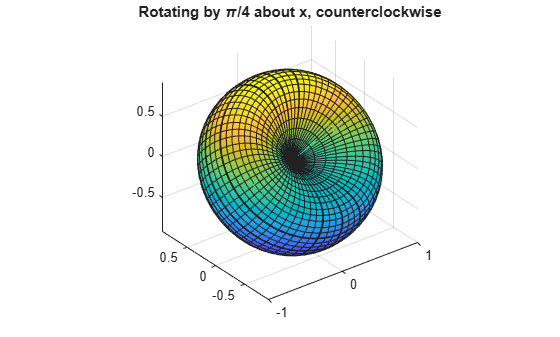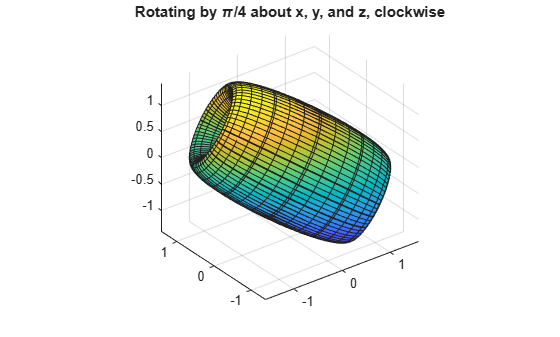Matrix Rotations and Transformations

This example shows how to do rotations and transforms in 3D using Symbolic Math Toolbox™ and matrices.

Define and Plot Parametric Surface

Define the parametric surface x(u,v), y(u,v), z(u,v) as follows.

syms u v
x = cos(u)*sin(v);
y = sin(u)*sin(v);
z = cos(v)*sin(v);

Plot the surface using fsurf.

fsurf(x,y,z)
axis equalCreate Rotation Matrices

Create 3-by-3 matrices Rx, Ry, and Rz representing plane rotations by an angle t about the x-, y-, and z-axis, respectively.

syms t

Rx = [1 0 0; 0 cos(t) -sin(t); 0 sin(t) cos(t)]
Rx =

$\left(\begin{array}{ccc}1& 0& 0\\ 0& \mathrm{cos}\left(t\right)& -\mathrm{sin}\left(t\right)\\ 0& \mathrm{sin}\left(t\right)& \mathrm{cos}\left(t\right)\end{array}\right)$

Ry = [cos(t) 0 sin(t); 0 1 0; -sin(t) 0 cos(t)]
Ry =

$\left(\begin{array}{ccc}\mathrm{cos}\left(t\right)& 0& \mathrm{sin}\left(t\right)\\ 0& 1& 0\\ -\mathrm{sin}\left(t\right)& 0& \mathrm{cos}\left(t\right)\end{array}\right)$

Rz = [cos(t) -sin(t) 0; sin(t) cos(t) 0; 0 0 1]
Rz =

$\left(\begin{array}{ccc}\mathrm{cos}\left(t\right)& -\mathrm{sin}\left(t\right)& 0\\ \mathrm{sin}\left(t\right)& \mathrm{cos}\left(t\right)& 0\\ 0& 0& 1\end{array}\right)$

Rotate About Each Axis in Three Dimensions

First, rotate the surface about the x-axis by 45 degrees counterclockwise.

xyzRx = Rx*[x;y;z];
Rx45 = subs(xyzRx, t, pi/4);

fsurf(Rx45(1), Rx45(2), Rx45(3))
title('Rotating by \pi/4 about x, counterclockwise')
axis equalRotate about the z-axis by 90 degrees clockwise.

xyzRz = Rz*Rx45;
Rx45Rz90 = subs(xyzRz, t, -pi/2);

fsurf(Rx45Rz90(1), Rx45Rz90(2), Rx45Rz90(3))
title('Rotating by \pi/2 about z, clockwise')
axis equalRotate about the y-axis by 45 degrees clockwise.

xyzRy = Ry*Rx45Rz90;
Rx45Rz90Ry45 = subs(xyzRy, t, -pi/4);

fsurf(Rx45Rz90Ry45(1), Rx45Rz90Ry45(2), Rx45Rz90Ry45(3))
title('Rotating by \pi/4 about y, clockwise')
axis equalScale and Rotate

Scale the surface by the factor 3 along the z-axis. You can multiply the expression for z by 3, z = 3*z. The more general approach is to create a scaling matrix, and then multiply the scaling matrix by the vector of coordinates.

S = [1 0 0; 0 1 0; 0 0 3];
xyzScaled = S*[x; y; z]
xyzScaled =

$\left(\begin{array}{c}\mathrm{cos}\left(u\right) \mathrm{sin}\left(v\right)\\ \mathrm{sin}\left(u\right) \mathrm{sin}\left(v\right)\\ 3 \mathrm{cos}\left(v\right) \mathrm{sin}\left(v\right)\end{array}\right)$

fsurf(xyzScaled(1), xyzScaled(2), xyzScaled(3))
title('Scaling by 3 along z')
axis equalRotate the scaled surface about the x-, y-, and z-axis by 45 degrees clockwise, in order z, then y, then x. The rotation matrix for this transformation is as follows.

R = Rx*Ry*Rz
R =

Use the rotation matrix to find the new coordinates.

xyzScaledRotated = R*xyzScaled;
xyzSR45 = subs(xyzScaledRotated, t, -pi/4);

Plot the surface.

fsurf(xyzSR45(1), xyzSR45(2), xyzSR45(3))
title('Rotating by \pi/4 about x, y, and z, clockwise')
axis equalCheck Properties of Rotation Matrix R

Rotation matrices are orthogonal matrices. Thus, the transpose of R is also its inverse, and the determinant of R is 1.

simplify(R.'*R)
ans =

$\left(\begin{array}{ccc}1& 0& 0\\ 0& 1& 0\\ 0& 0& 1\end{array}\right)$

simplify(det(R))
ans = $1$

Symbolic Math Toolbox Documentation

Mathematical Modeling with Symbolic Math Toolbox

Get examples and videos